Most Affordable JEE | NEET | 8,9,10 Preparation by Kota's Top IITian Doctor Faculties

# NCERT Solutions for Class 9 Science chapter 9 - Force and Laws of Motion - eSaral

Class 9Hey, are you a class 9 student and looking for ways to download NCERT Solutions for Class 9 Science Chapter 9 "Force and Laws of Motion"? If yes. Then you are at the right place.

In this article, we have listed NCERT solutions for class 9 Science Physics Chapter 9 in PDF that is prepared by Kota’s top IITian’s Faculties by keeping Simplicity in mind.

If you want to learn and understand class 9 Science chapter 9 "Force and Laws of Motion" in an easy way then you can use these solutions PDF.

NCERT Solutions helps students to Practice important concepts of subjects easily. Class 9 Science solutions provide detailed explanations of all the NCERT exercise questions that students can use to clear their doubts instantly.

If you want to score high in your class 9 Science Exam then it is very important for you to have a good knowledge of all the important topics, so to learn and practice those topics you can use eSaral NCERT Solutions.

So, without wasting more time Let’s start.

### Download The PDF of NCERT Solutions for Class 9 Science Chapter 9 "Force and Laws of Motion"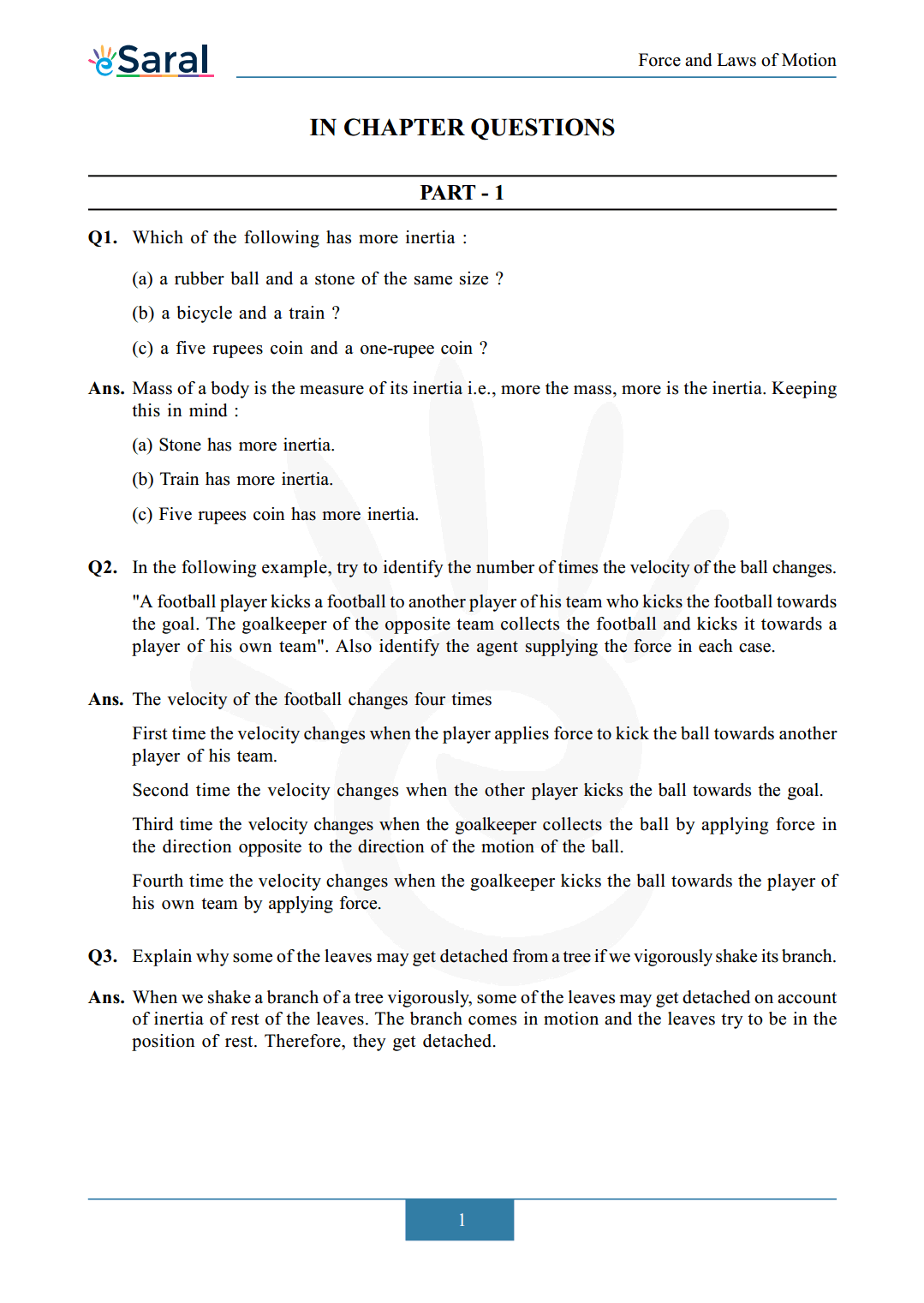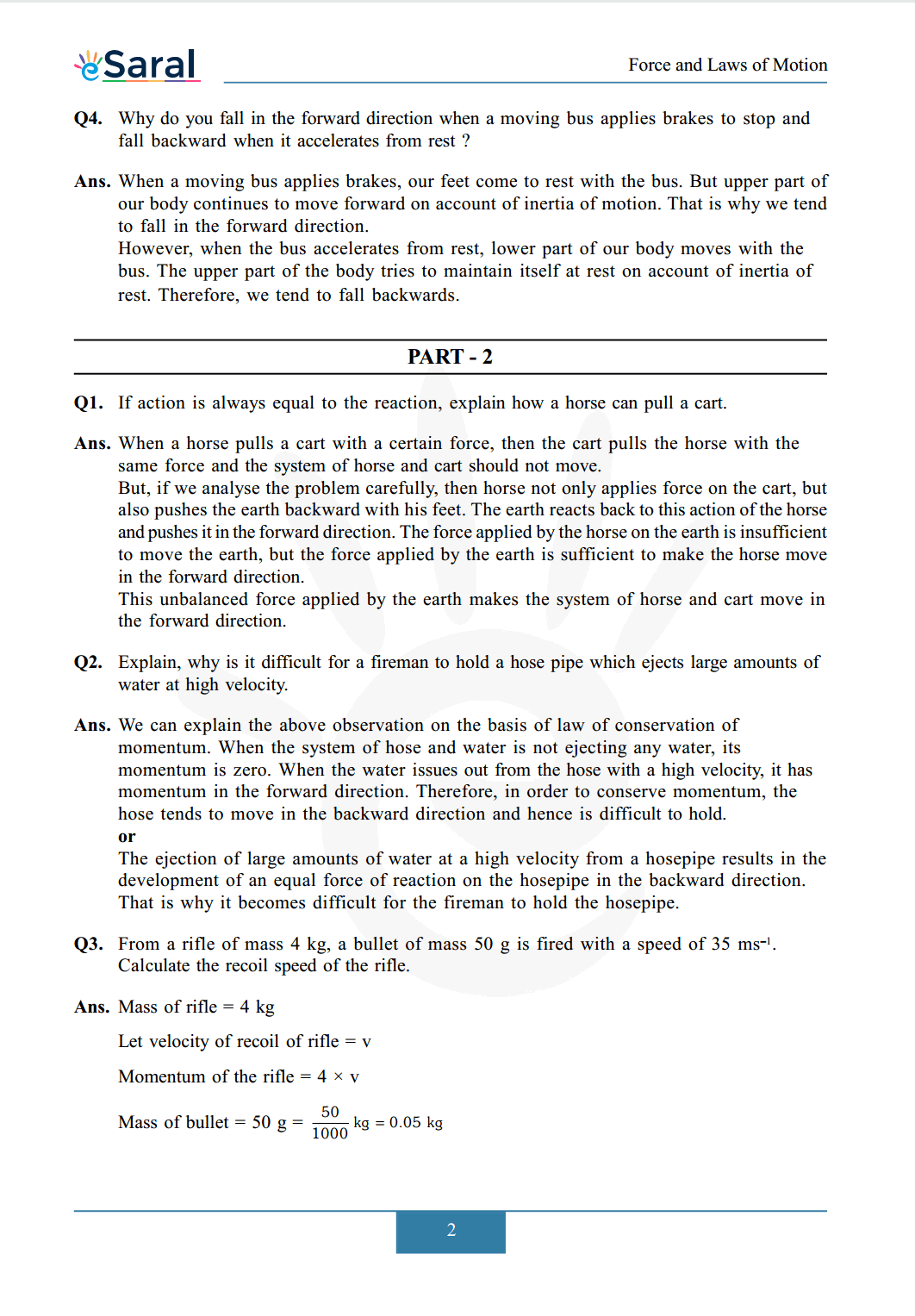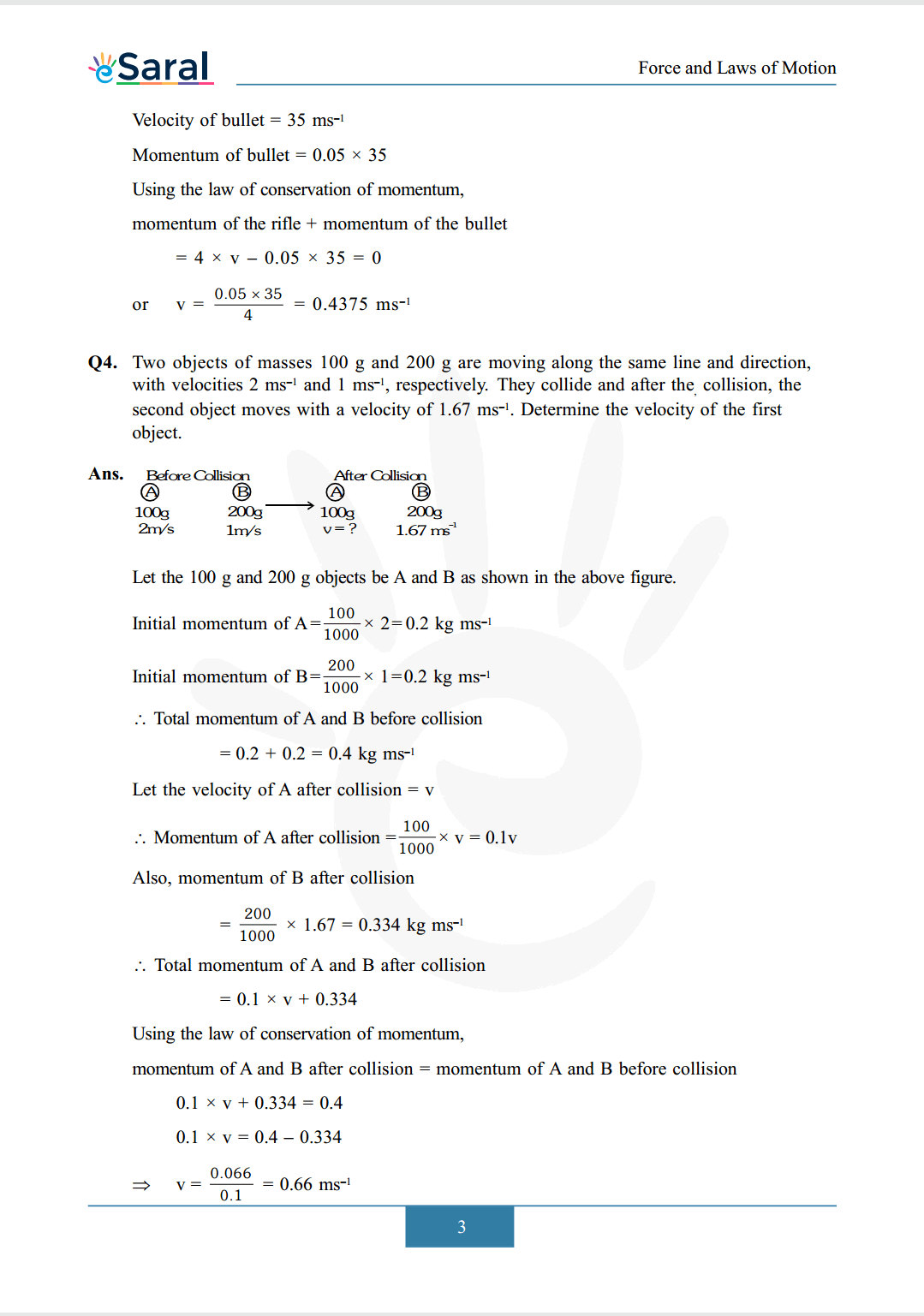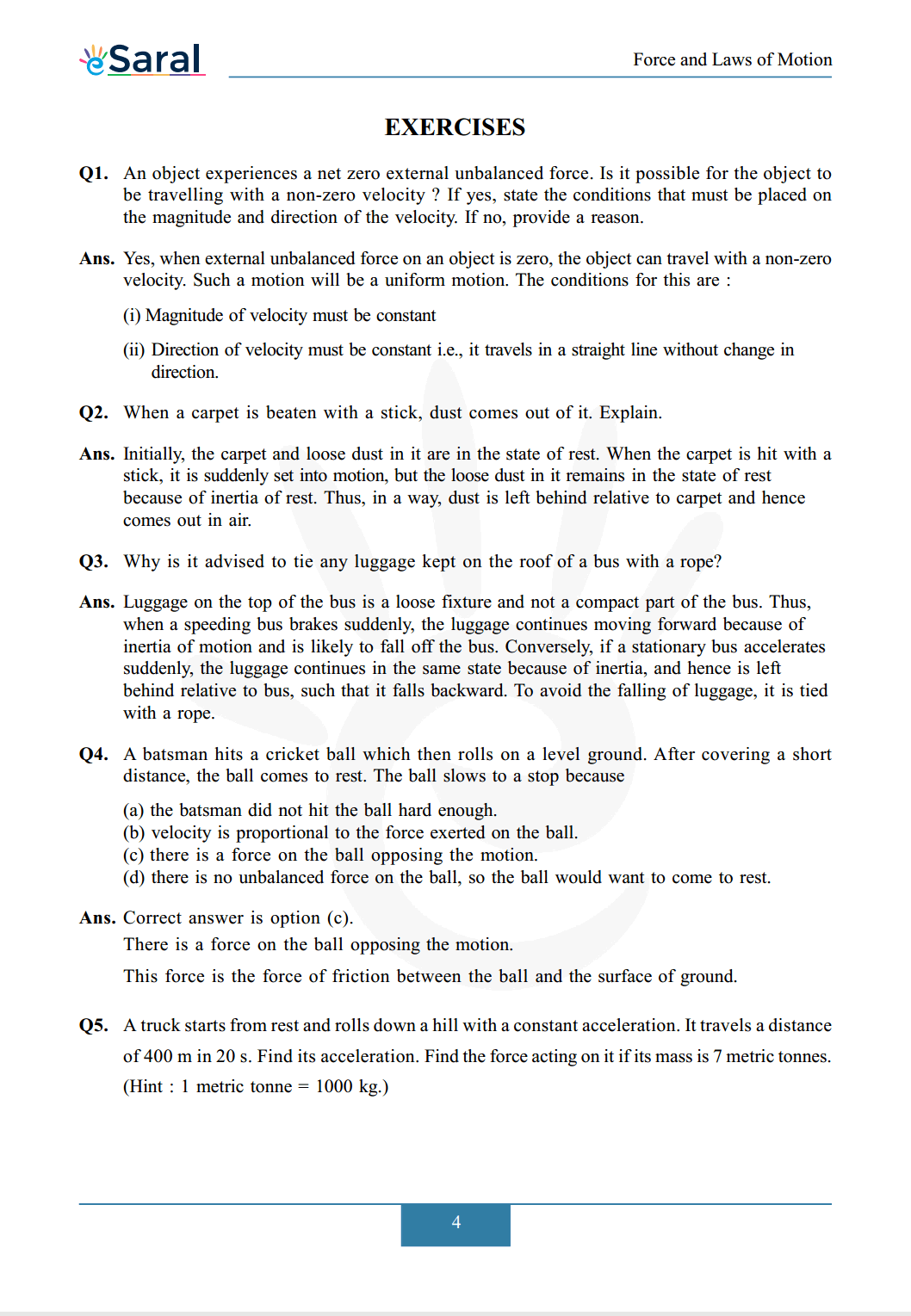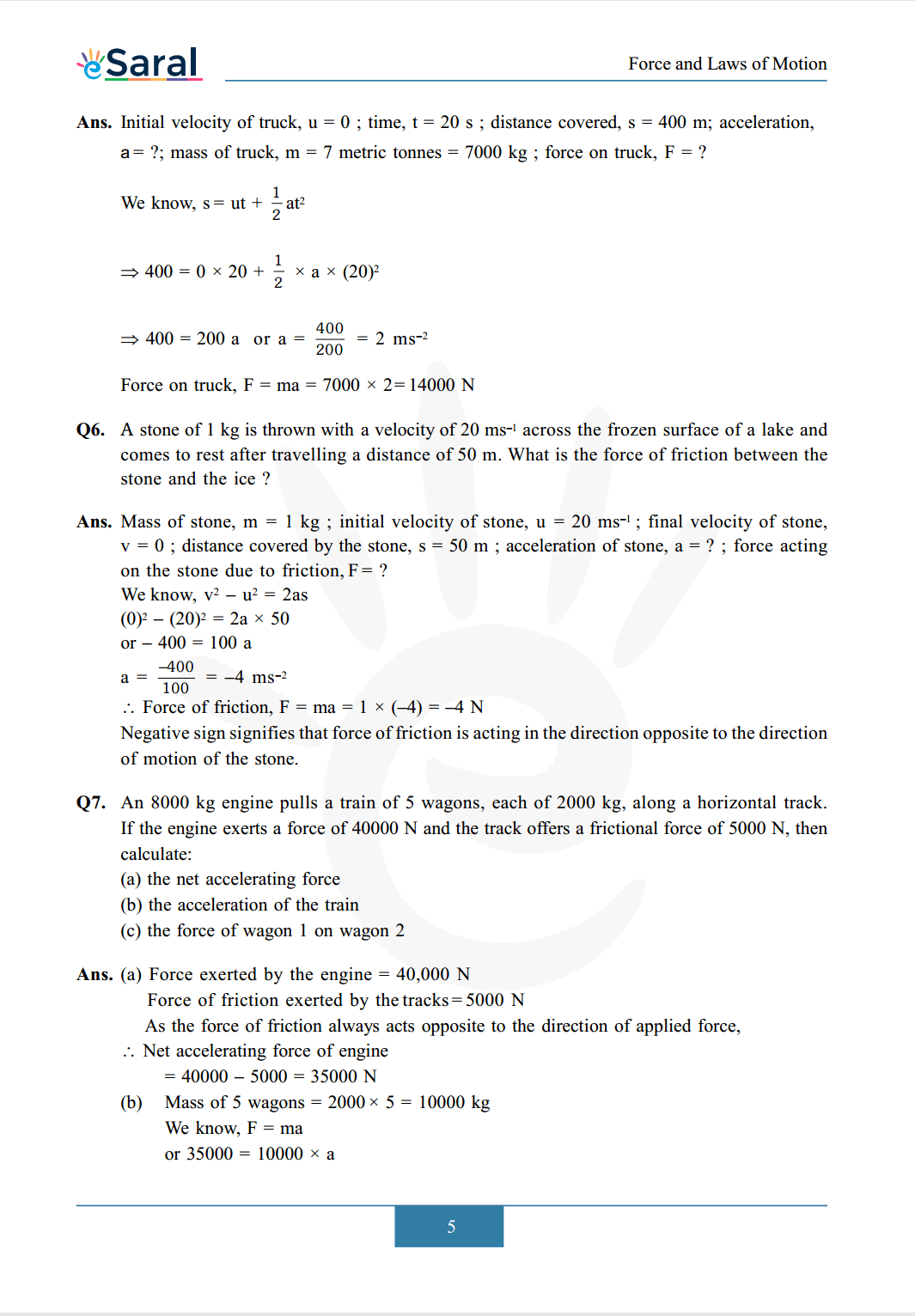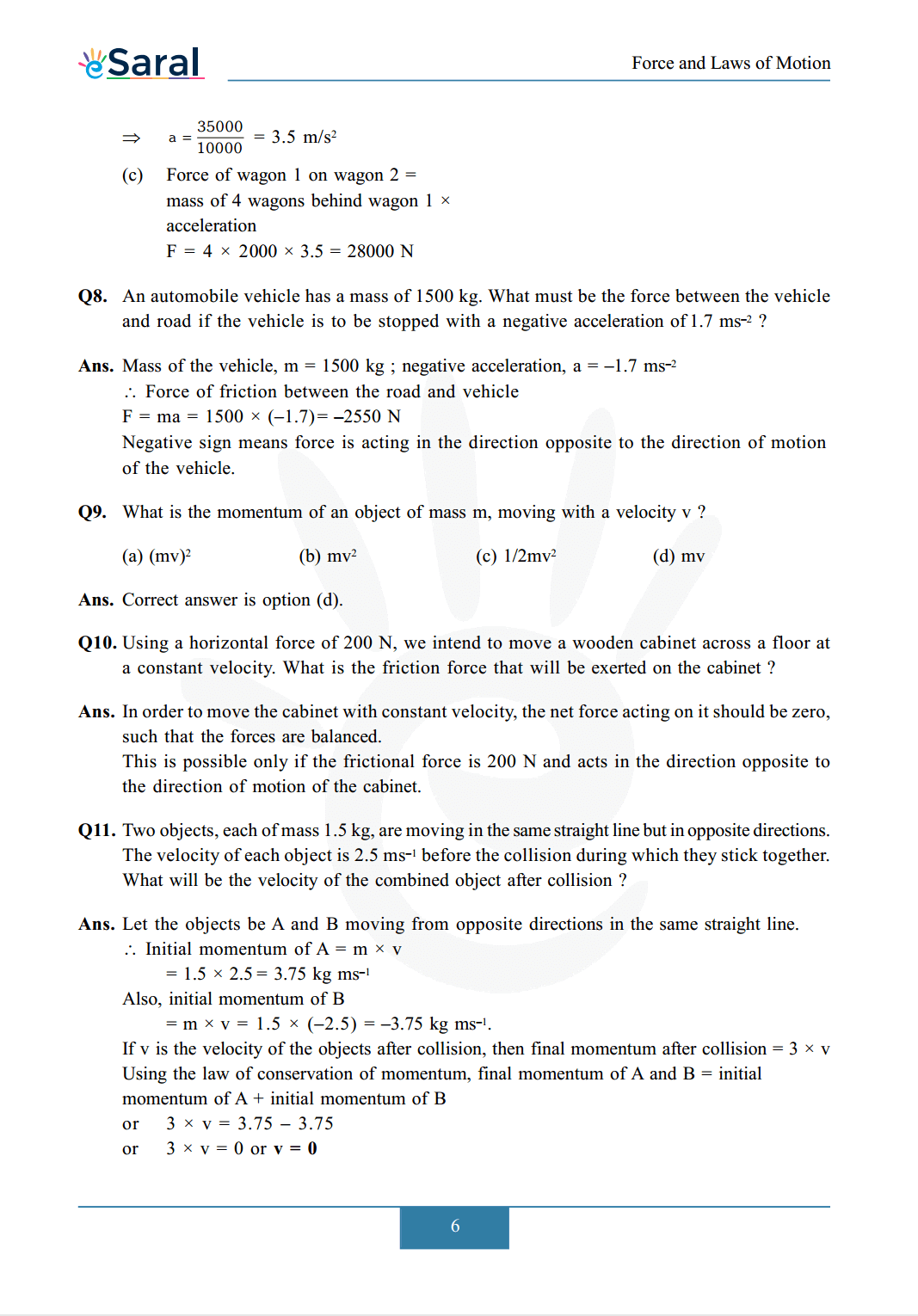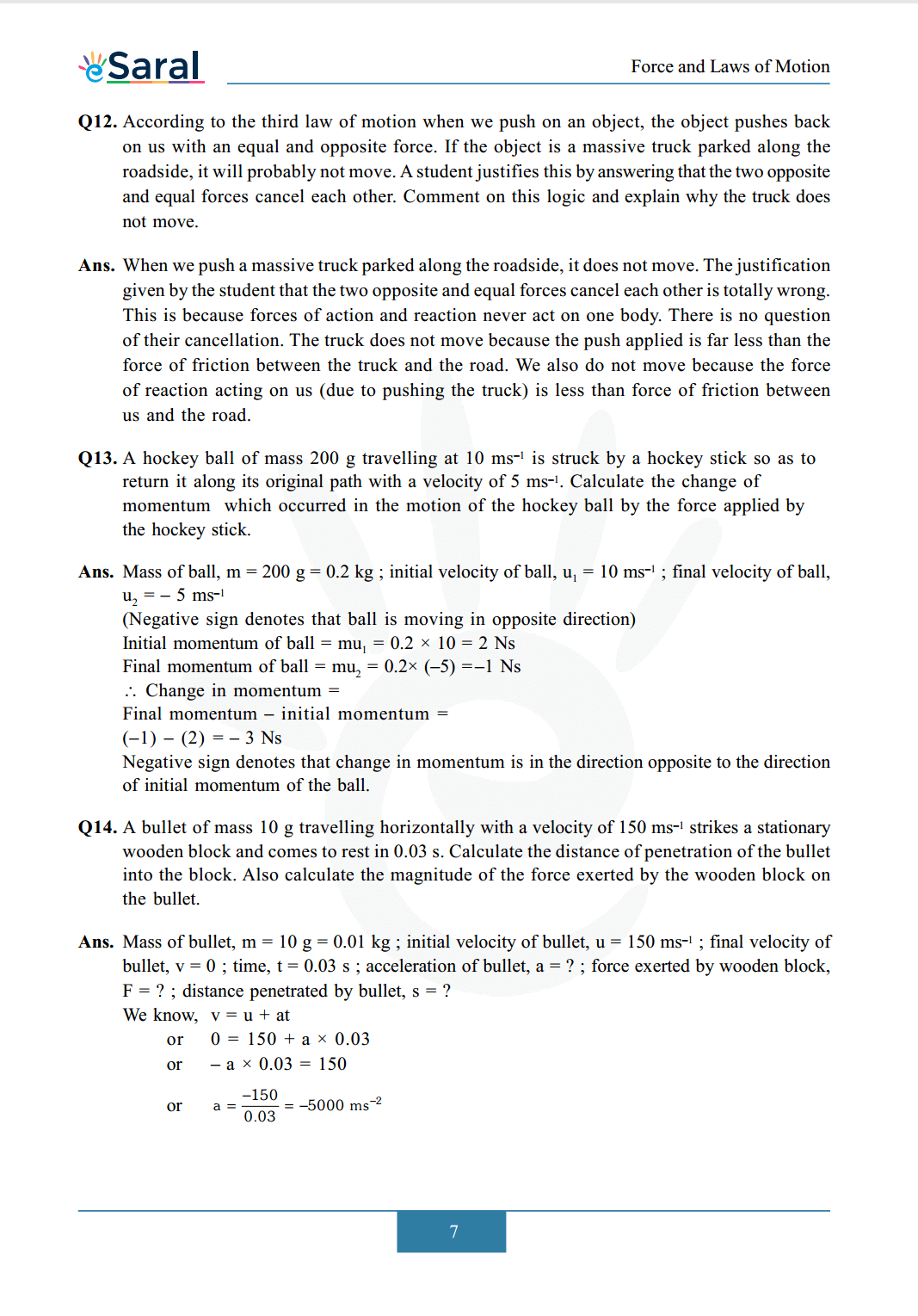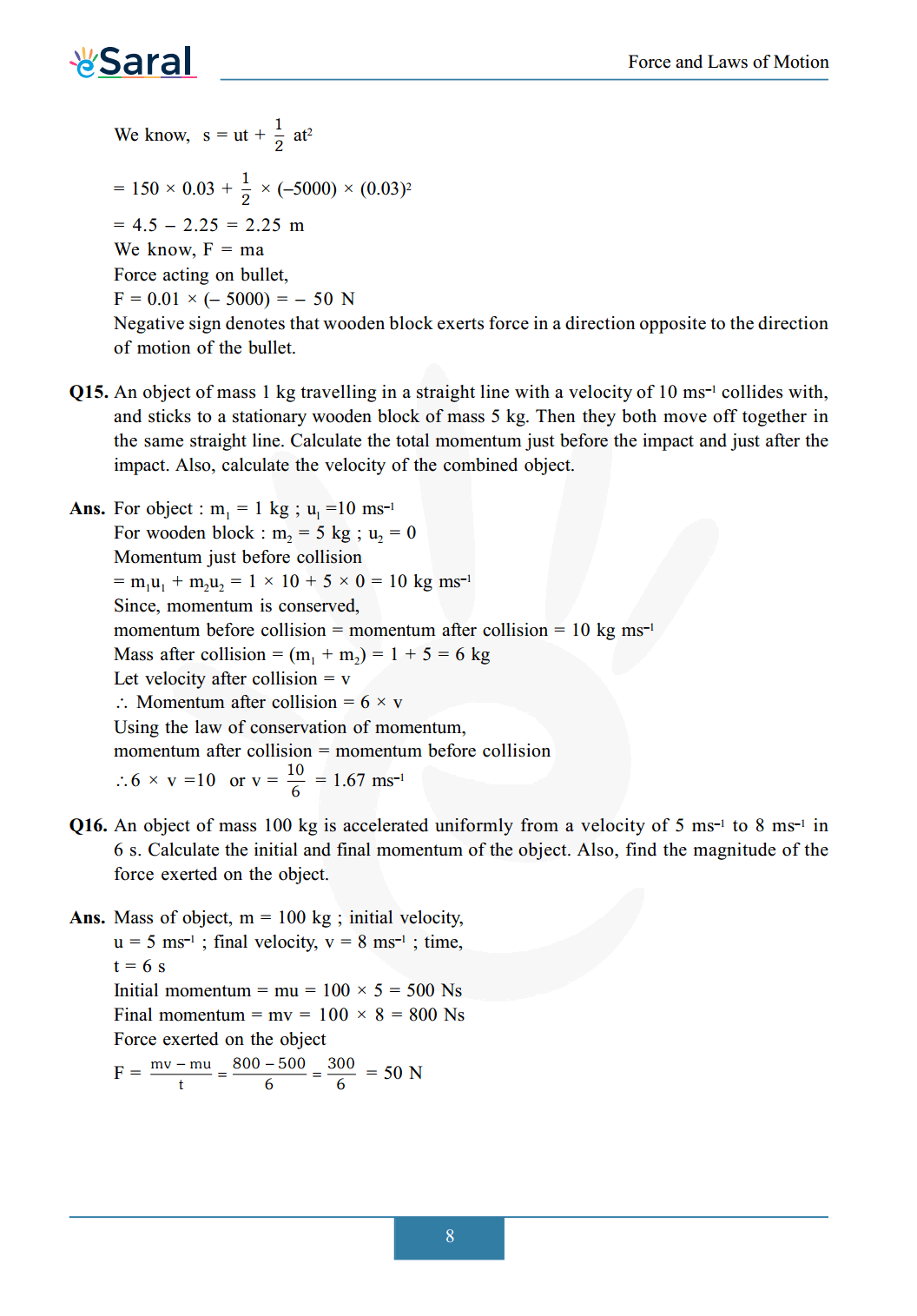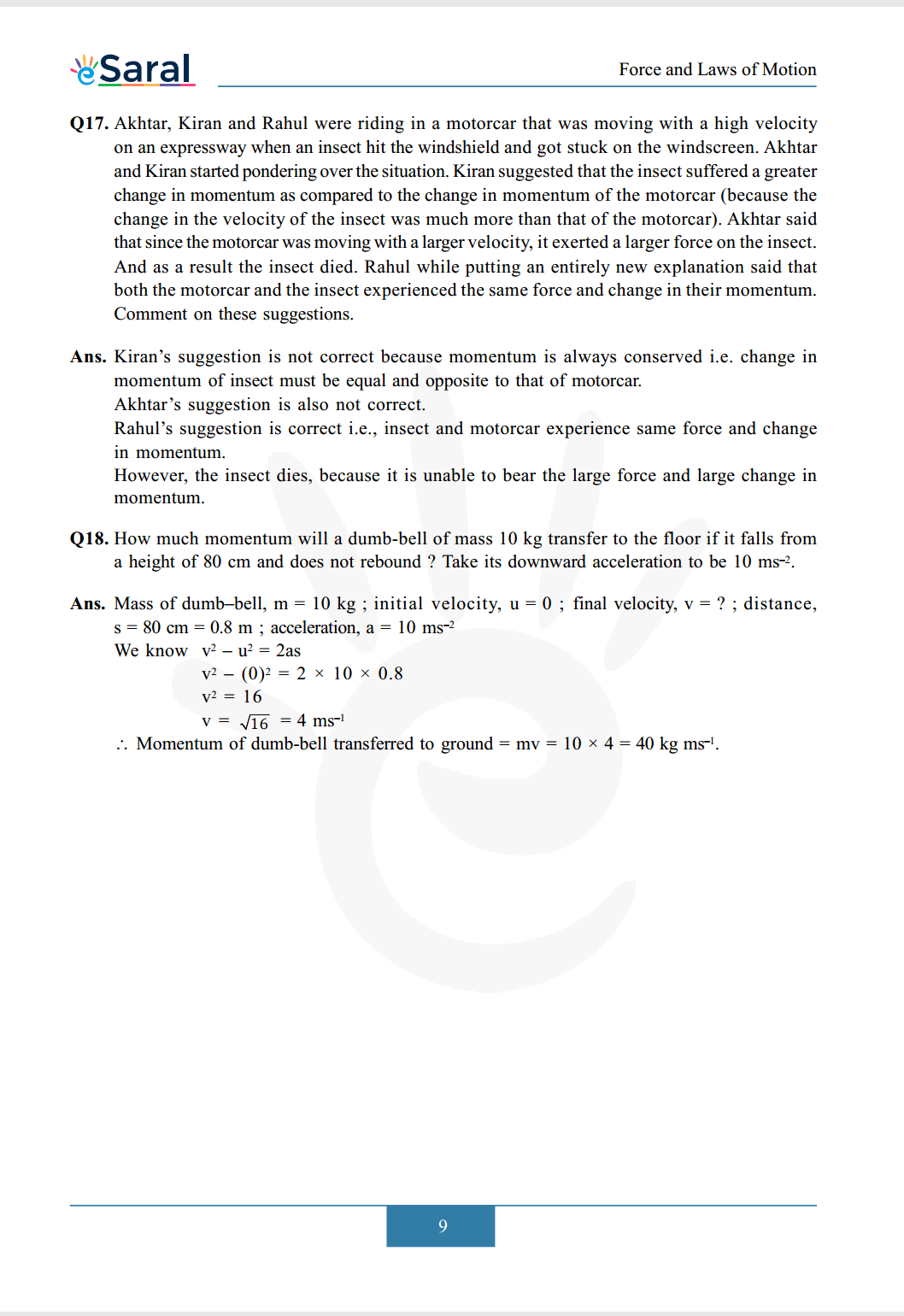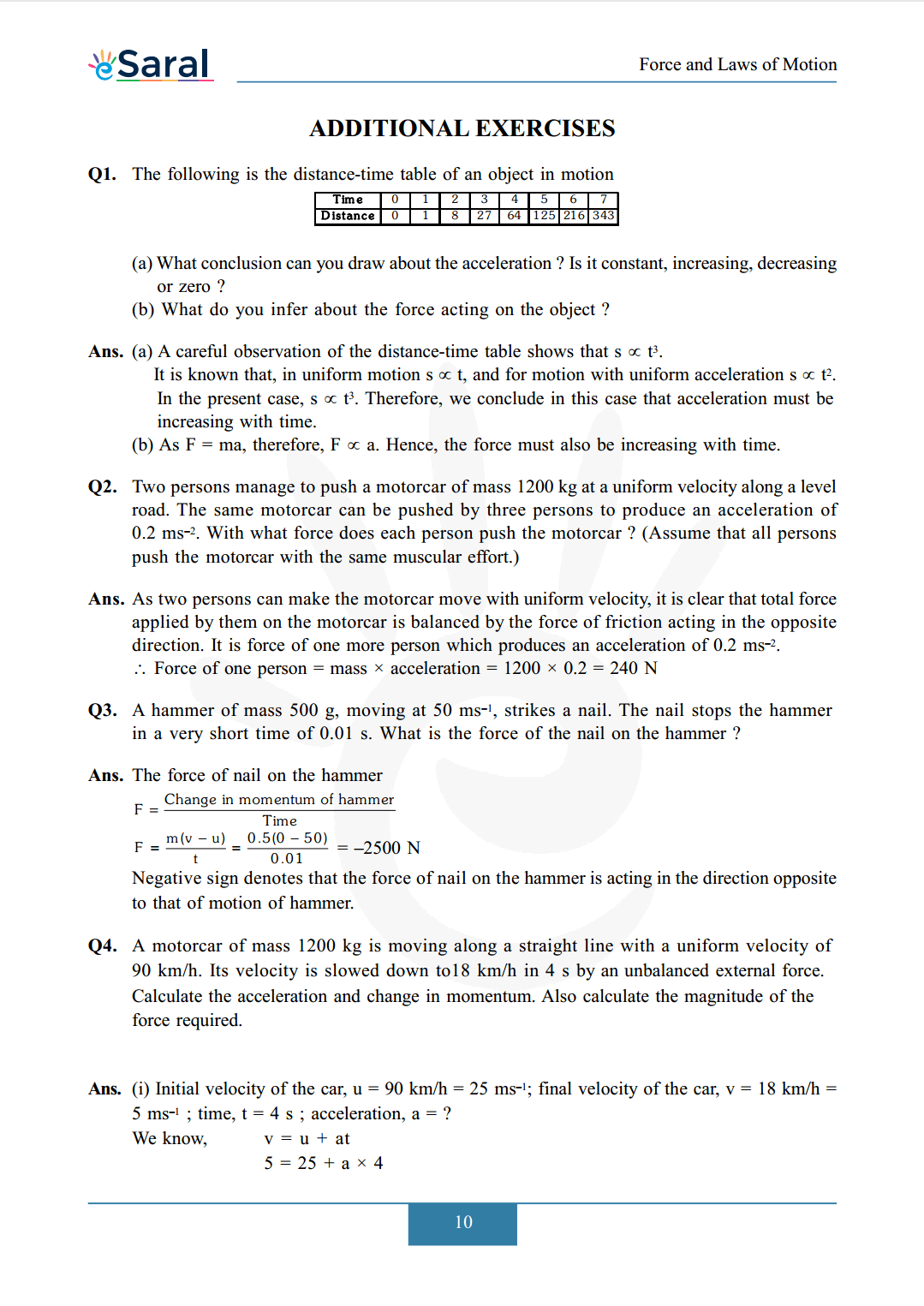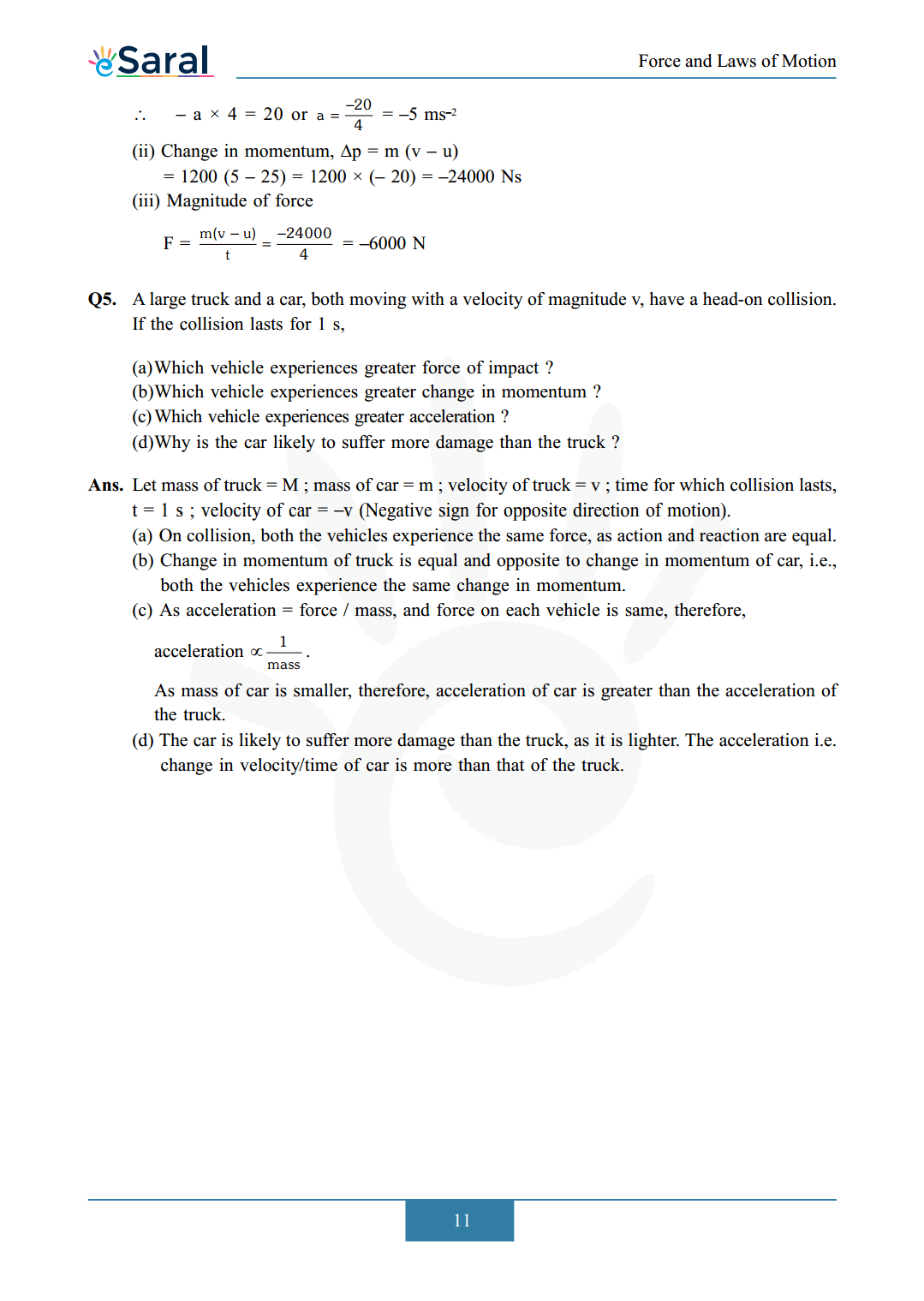Download The PDF of NCERT Solutions for Class 9 Science Chapter 9 "Force and Laws of Motion"

### Important topics of class 9 Science Chapter 9

• Force and Laws Of Motion
• Balanced and Unbalanced force
• Inertia and Mass
• First Law of Motion
• Second Law of Motion
• Third Law of Motion
• Conservation of Momentum

#### All Questions of Chapter 9

Once you complete the chapter 9 then you can revise it by solving following questions.

Q1. Which of the following has more inertia :
(a) a rubber ball and a stone of the same size ?
(b) a bicycle and a train ?
(c) a five rupees coin and a one-rupee coin ?

Q2. In the following example, try to identify the number of times the velocity of the ball changes.
"A football player kicks a football to another player of his team who kicks the football towards the goal. The goalkeeper of the opposite team collects the football and kicks it towards a player of his own team". Also identify the agent supplying the force in each case.

Q3. Explain why some of the leaves may get detached from a tree if we vigorously shake its branch.

Q4. Why do you fall in the forward direction when a moving bus applies brakes to stop and fall backward when it accelerates from rest ?

##### Part 2

Q1. If action is always equal to the reaction, explain how a horse can pull a cart.

Q2. Explain, why is it difficult for a fireman to hold a hose pipe which ejects large amounts of water athigh velocity.

Q3. From a rifle of mass $4 \mathrm{~kg}$, a bullet of mass $50 \mathrm{~g}$ is fired with a speed of $35 \mathrm{~ms}^{-1}$. Calculate the recoil speed of the rifle.

Q4. Two objects of masses $100 \mathrm{~g}$ and $200 \mathrm{~g}$ are moving along the same line and direction, with velocities $2 \mathrm{~ms}^{-1}$ and $1 \mathrm{~ms}^{-1}$, respectively. They collide and after the collision, the second object moves with a velocity of $1.67 \mathrm{~ms}^{-1}$. Determine the velocity of the first object.

##### Exercise

Q1. An object experiences a net zero external unbalanced force. Is it possible for the object to be travelling with a non-zero velocity ? If yes, state the conditions that must be placed on the magnitude and direction of the velocity. If no, provide a reason.

Q2. When a carpet is beaten with a stick, dust comes out of it. Explain.

Q3. Why is it advised to tie any luggage kept on the roof of a bus with a rope?

Q4. A batsman hits a cricket ball which then rolls on a level ground. After covering a short distance, the ball comes to rest. The ball slows to a stop because
(a) the batsman did not hit the ball hard enough.
(b) velocity is proportional to the force exerted on the ball.
(c) there is a force on the ball opposing the motion.
(d) there is no unbalanced force on the ball, so the ball would want to come to rest.

Q5. A truck starts from rest and rolls down a hill with a constant acceleration. It travels a distance of $400 \mathrm{~m}$ in $20 \mathrm{~s}$. Find its acceleration. Find the force acting on it if its mass is 7 metric tonnes. (Hint : 1 metric tonne $=1000 \mathrm{~kg} .$ )

Q6. A stone of $1 \mathrm{~kg}$ is thrown with a velocity of $20 \mathrm{~ms}^{-1}$ across the frozen surface of a lake and comes to rest after travelling a distance of $50 \mathrm{~m}$. What is the force of friction between the stone and the ice ?

Q7. An $8000 \mathrm{~kg}$ engine pulls a train of 5 wagons, each of $2000 \mathrm{~kg}$, along a horizontal track. If the engine exerts a force of $40000 \mathrm{~N}$ and the track offers a frictional force of $5000 \mathrm{~N}$, then calculate:
(a) the net accelerating force
(b) the acceleration of the train
(c) the force of wagon 1 on wagon 2

Q8. An automobile vehicle has a mass of $1500 \mathrm{~kg}$. What must be the force between the vehicle and road if the vehicle is to be stopped with a negative acceleration of $1.7 \mathrm{~ms}^{-2}$ ?

Q9. What is the momentum of an object of mass m, moving with a velocity v ?
(a) $(\mathrm{mv})^{2}$
(b) $\mathrm{mv}^{2}$
(c) $1 / 2 \mathrm{mv}^{2}$
(d) $\mathrm{mv}$

Q10. Using a horizontal force of $200 \mathrm{~N}$, we intend to move a wooden cabinet across a floor at a constant velocity. What is the friction force that will be exerted on the cabinet?

Q11. Two objects, each of mass $1.5 \mathrm{~kg}$, are moving in the same straight line but in opposite directions. The velocity of each object is $2.5 \mathrm{~ms}^{-1}$ before the collision during which they stick together. What will be the velocity of the combined object after collision?

Q12. According to the third law of motion when we push on an object, the object pushes back on us with an equal and opposite force. If the object is a massive truck parked along the roadside, it will probably not move. A student justifies this by answering that the two opposite and equal forces cancel each other. Comment on this logic and explain why the truck does not move.

Q13. A hockey ball of mass $200 \mathrm{~g}$ travelling at $10 \mathrm{~ms}^{-1}$ is struck by a hockey stick so as to return it along its original path with a velocity of $5 \mathrm{~ms}^{-1} .$ Calculate the change of momentum which occurred in the motion of the hockey ball by the force applied by the hockey stick.

Q14. A bullet of mass $10 \mathrm{~g}$ travelling horizontally with a velocity of $150 \mathrm{~ms}^{-1}$ strikes a stationary wooden block and comes to rest in $0.03 \mathrm{~s}$. Calculate the distance of penetration of the bullet into the block. Also calculate the magnitude of the force exerted by the wooden block on the bullet.

Q15. An object of mass $1 \mathrm{~kg}$ travelling in a straight line with a velocity of $10 \mathrm{~ms}^{-1}$ collides with, and sticks to a stationary wooden block of mass $5 \mathrm{~kg}$. Then they both move off together in the same straight line. Calculate the total momentum just before the impact and just after the impact. Also, calculate the velocity of the combined object.

Q16. An object of mass $100 \mathrm{~kg}$ is accelerated uniformly from a velocity of $5 \mathrm{~ms}^{-1}$ to $8 \mathrm{~ms}^{-1}$ in 6 s. Calculate the initial and final momentum of the object. Also, find the magnitude of the force exerted on the object.

Q17. Akhtar, Kiran and Rahul were riding in a motorcar that was moving with a high velocity on an expressway when an insect hit the windshield and got stuck on the windscreen. Akhtar and Kiran started pondering over the situation. Kiran suggested that the insect suffered a greater change in momentum as compared to the change in momentum of the motorcar (because the change in the velocity of the insect was much more than that of the motorcar). Akhtar said that since the motorcar was moving with a larger velocity, it exerted a larger force on the insect. And as a result the insect died. Rahul while putting an entirely new explanation said that both the motorcar and the insect experienced the same force and change in their momentum. Comment on these suggestions.

Q18. How much momentum will a dumb-bell of mass $10 \mathrm{~kg}$ transfer to the floor if it falls from a height of $80 \mathrm{~cm}$ and does not rebound ? Take its downward acceleration to be $10 \mathrm{~ms}^{-2}$.

Q1. The following is the distance-time table of an object in motion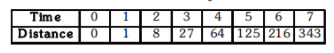Q2. Two persons manage to push a motorcar of mass $1200 \mathrm{~kg}$ at a uniform velocity along a level road. The same motorcar can be pushed by three persons to produce an acceleration of $0.2 \mathrm{~ms}^{-2}$. With what force does each person push the motorcar? (Assume that all persons push the motorcar with the same muscular effort.)

Q3. A hammer of mass $500 \mathrm{~g}$, moving at $50 \mathrm{~ms}^{-1}$, strikes a nail. The nail stops the hammer in a very short time of $0.01 \mathrm{~s}$. What is the force of the nail on the hammer?

Q4. A motorcar of mass $1200 \mathrm{~kg}$ is moving along a straight line with a uniform velocity of $90 \mathrm{~km} / \mathrm{h}$. Its velocity is slowed down to $18 \mathrm{~km} / \mathrm{h}$ in $4 \mathrm{~s}$ by an unbalanced external force. Calculate the acceleration and change in momentum. Also calculate the magnitude of the force required.

Q5. A large truck and a car, both moving with a velocity of magnitude $\mathrm{v}$, have a head-on collision. If the collision lasts for $1 \mathrm{~s}$,
(a)Which vehicle experiences greater force of impact ?
(b)Which vehicle experiences greater change in momentum ?
(c)Which vehicle experiences greater acceleration ?
(d)Why is the car likely to suffer more damage than the truck ?